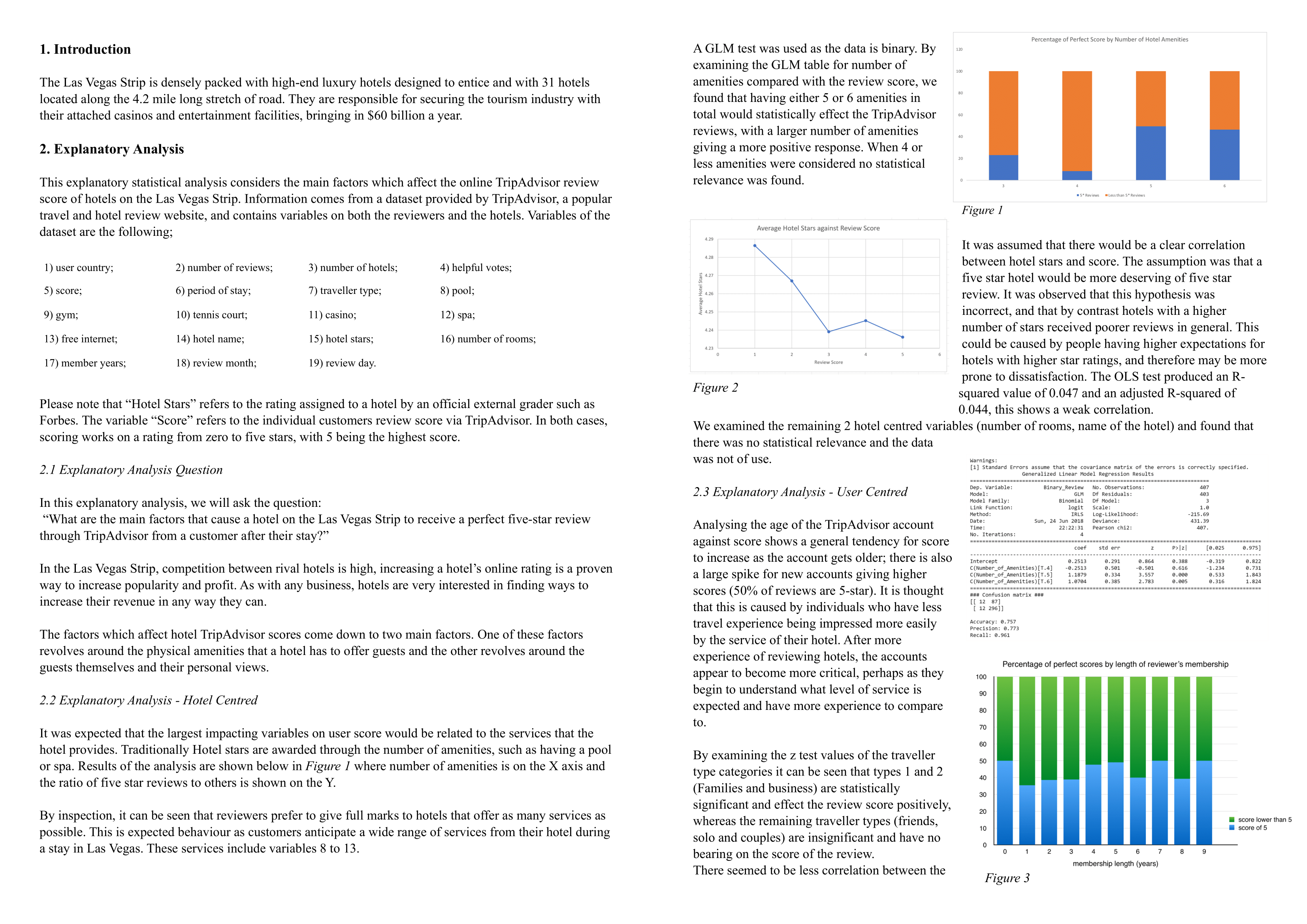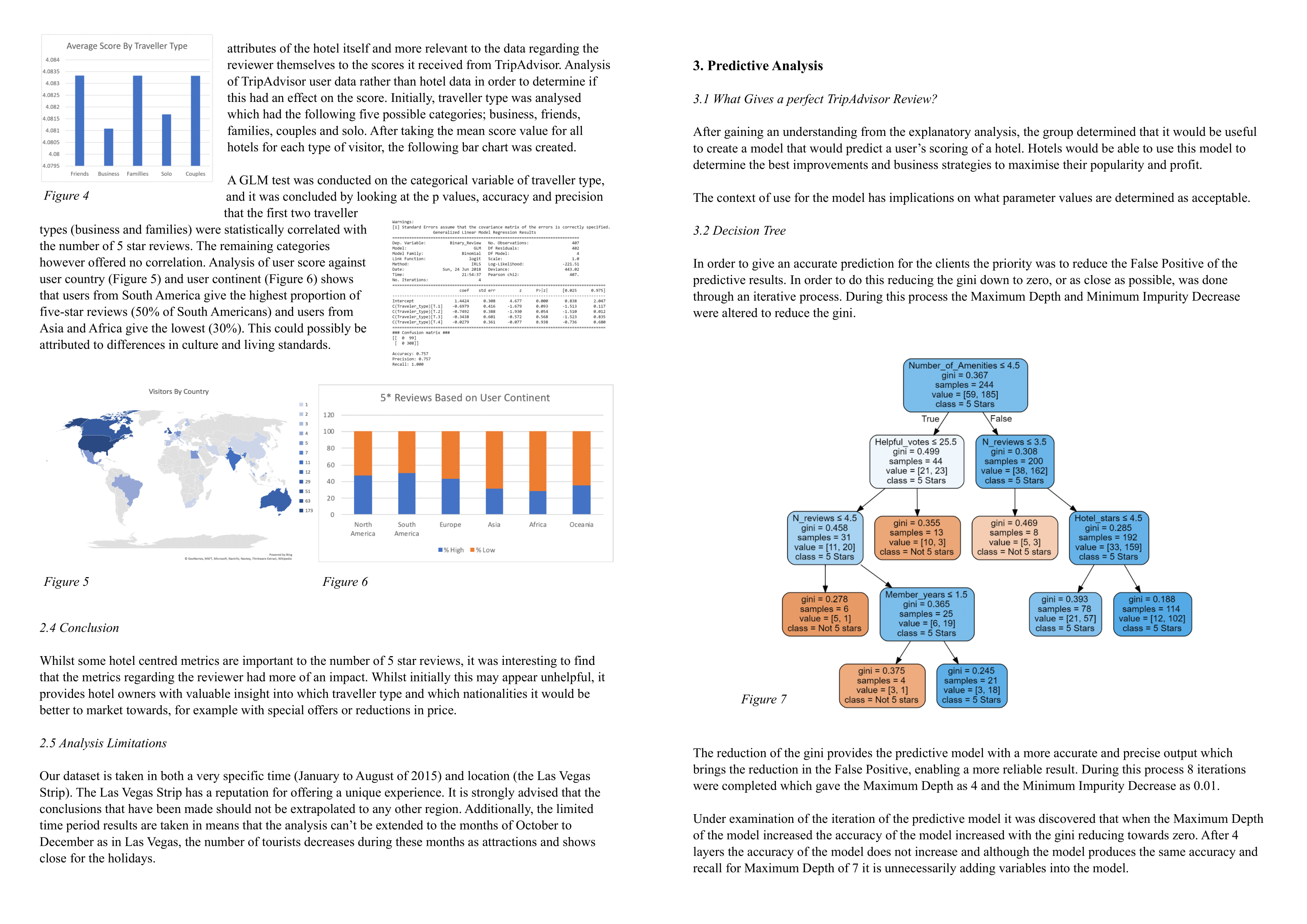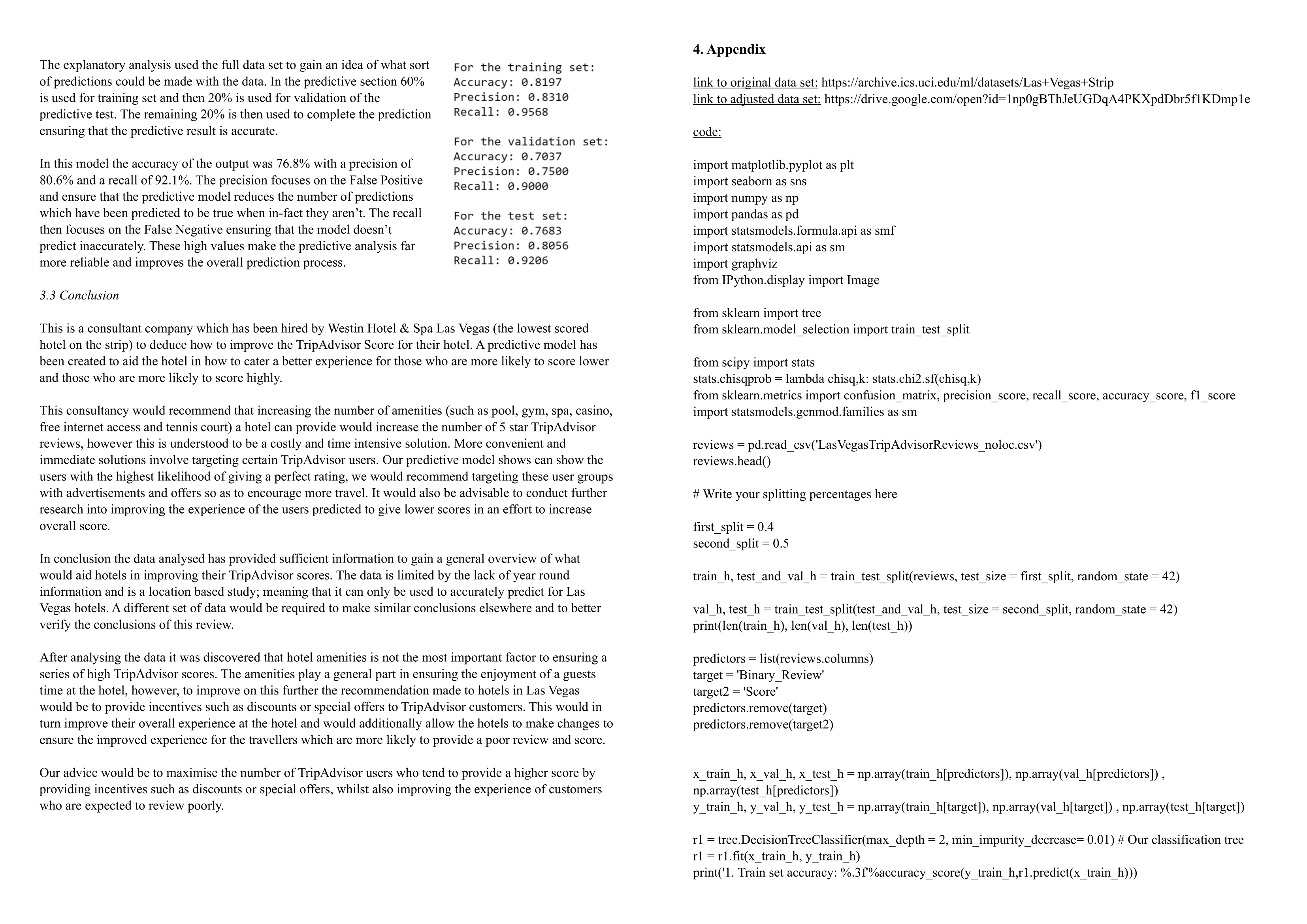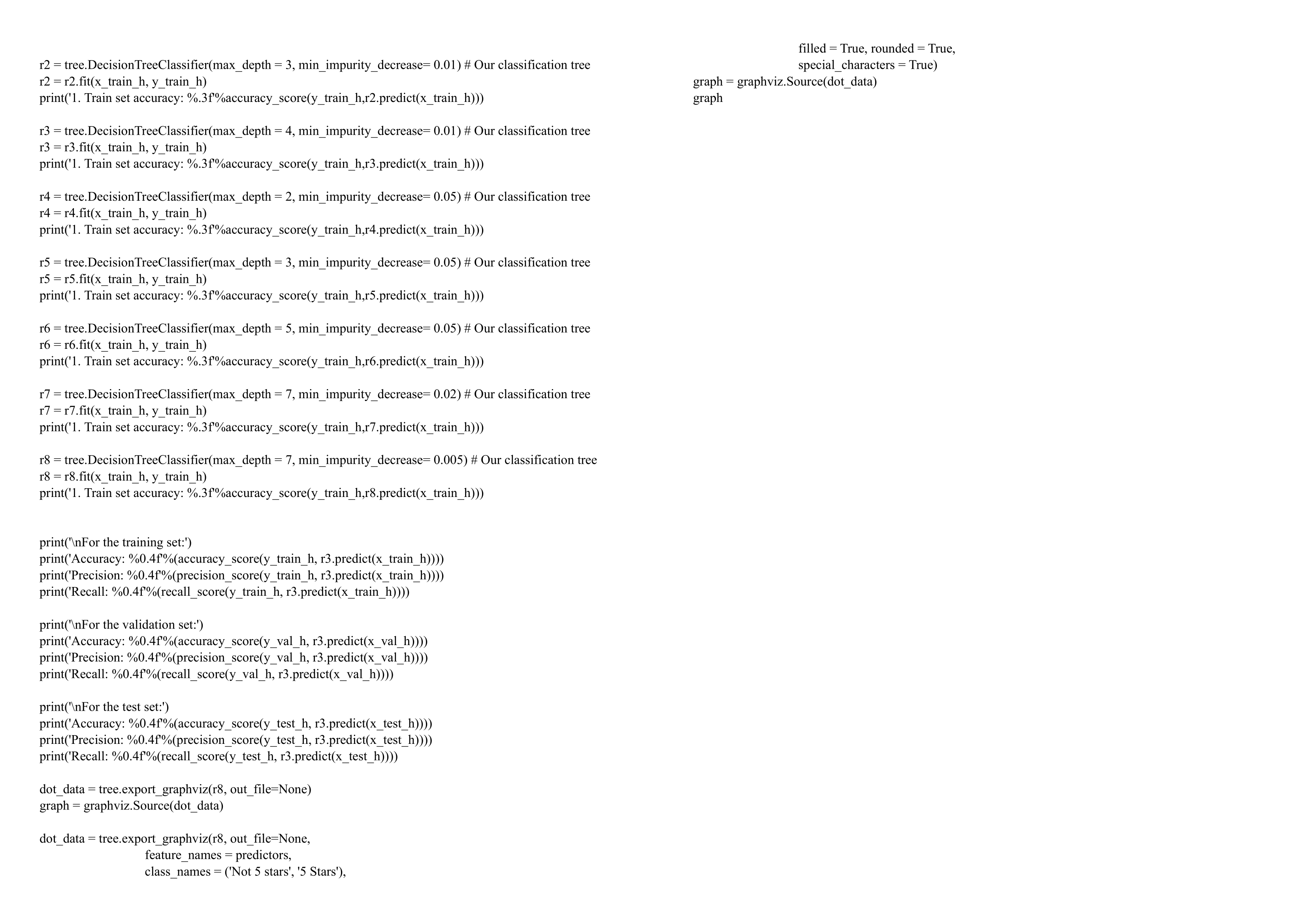﻿ Bigtg Data

# Big Data

## Data Analysis

• I conducted an explanatory and predictive analysis on a dataset using machine learning with Python, this involved cleaning, reducing and transforming the data set and useing a non-linear decision tree classifier with an accuracy, precision and recall of 70%, 75% and 90%.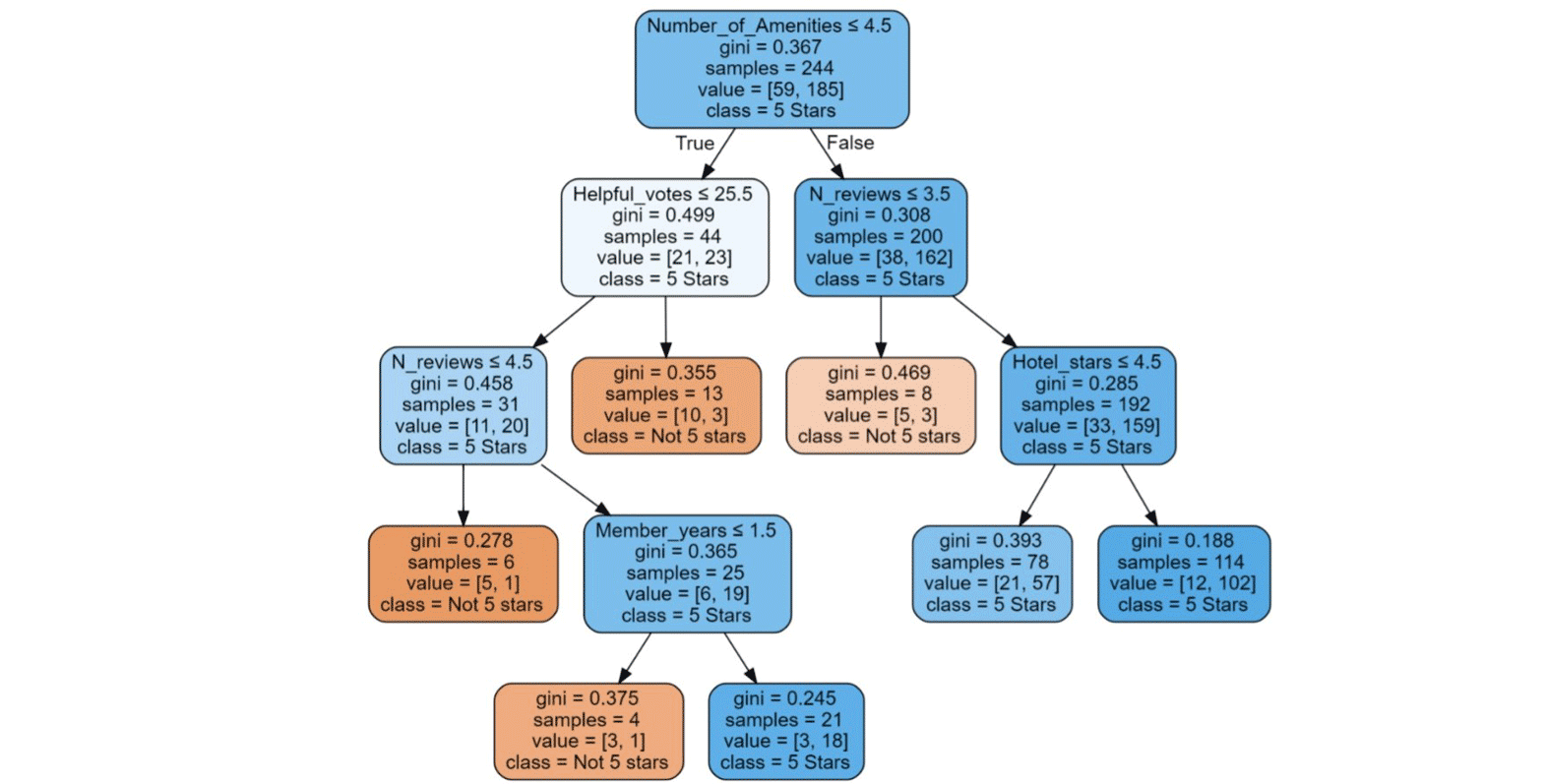## Method

We sourced a data set about Las Vegas hotels in order to find out what factors affected hotel ratings. We used general linear regression to identify which of the categorical factors had the moset effect on a hotel's score. The dataset had 20 features.

```glm_binom = sm.GLM(data.endog, data.exog, family=sm.families.Binomial())
res = glm_binom.fit()```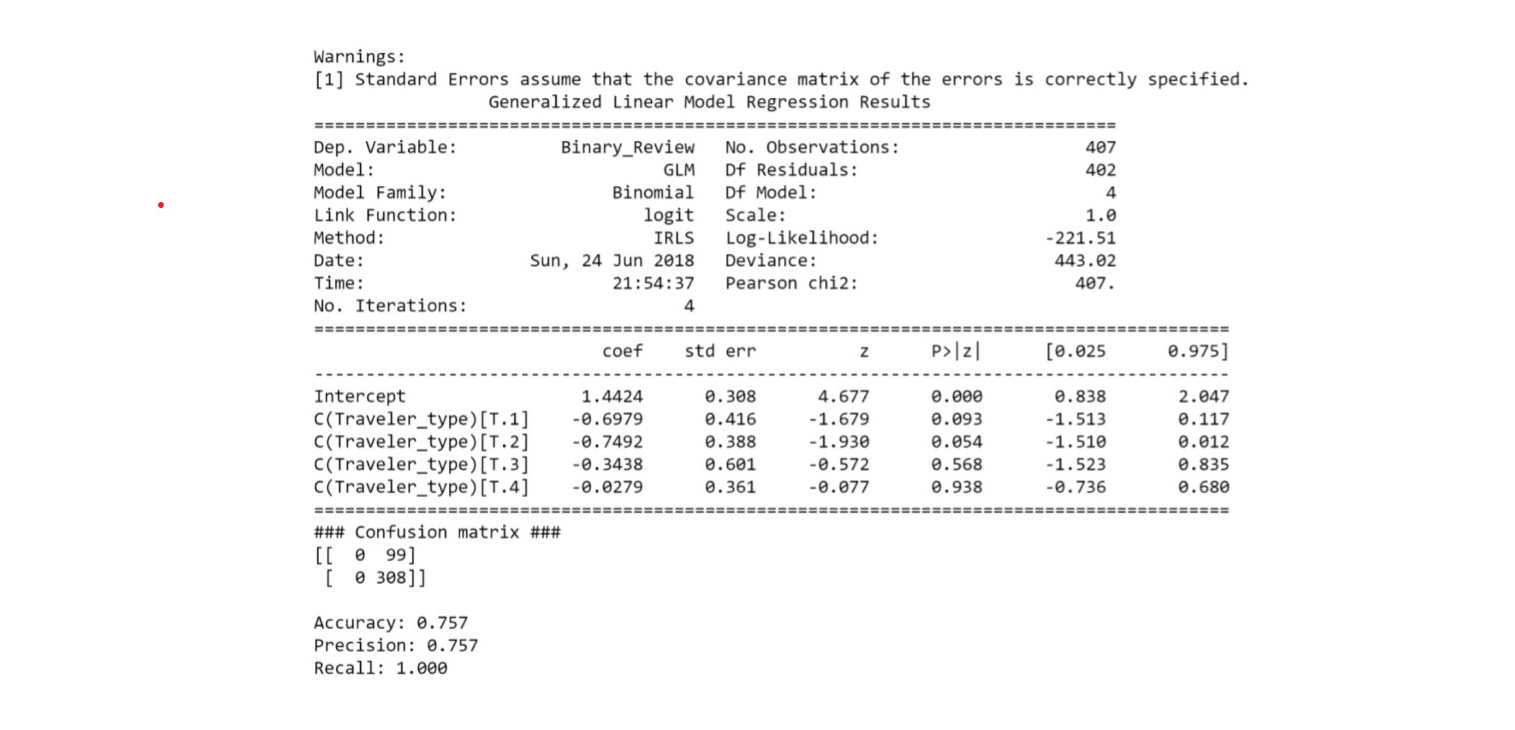A A non linear decision tree was implemented through 8 iterations. This gave the Maximum Depth as 4 and the Minimum Impurity Decrease as 0.01.

```x_train_h, x_val_h, x_test_h = np.array(train_h[predictors]), np.array(val_h[predictors]) ,
np.array(test_h[predictors])
y_train_h, y_val_h, y_test_h = np.array(train_h[target]), np.array(val_h[target]) , np.array(test_h[target])

r8 = tree.DecisionTreeClassifier(max_depth = 7, min_impurity_decrease= 0.005) # Our classification tree
r8 = r8.fit(x_train_h, y_train_h)
print('1. Train set accuracy: %.3f'%accuracy_score(y_train_h,r8.predict(x_train_h)))```

### Report